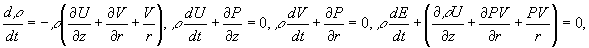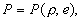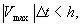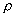Computational experiment technology

V.F.Minin, I.V.Minin, O.V.Minin

The basic aspects of the technology of the computational experiment (CE), principles of program-technical systems (PTS) construction, developed under the scientific leadership of Professor Vladilen F. Minin at the Institute of Applied Physics (Novosibirsk, USSR) on the basic of experience of development and usage of similar systems for 25 years and designed for realization of mass computational experiment are formulated in the given work. The main parameters of PTS are yielded, some most interesting trends of CE conduction are shown, each of them is illustrated with the relevant solved problems.

The development of the mathematical physics, computational mathematics and means of computer technology gives the possibility to analyze the processes of pulse action upon a substance by conducting the computational experiment. It should be noted that in a general case the notion of CE is wider than the notion of simple modulation. The main difference is in the fact that CE makes possible to answer the question: "What will be if ...?"

On the basis of experience of development and usage of PTS the following main principles of PTS construction were formulated [1,2]:

- the problem solution can be carried out by a researcher who is not a specialist in the region of numerical methods and programming;

- PTS operate in a dialogue regime in terms of a researcher;

- all the initial and resulting information is represented in a convenient from analysis and displayed, that is the principle of "no dumps" is realized;

The PTS satisfying the requirements given above was realized using two 64-processor computers on the basic of the multiprocessor PS-2000 and a serial graphic display station of "Gamma" series  developed at the Institute of Applied Physics under the scientific leadership of Professor Vladilen F. Minin. For complete realization of multiprocessor possibilities a special code allowing to carry out its paralleling was developed to solve the equation of continuum mechanics. For the solution of a wide class of problems besides generally used mathematical models of description of material and substance properties the wide-range equations of state of metals and description models of nonstationary detonation of heterogeneous explosives taking into account the kinetics of their decomposition in shock waves were included in PTS.

The main parameters of PTS are as follows: the RAM capacity is 2000000 24-digit words, the calculation field size is to 200000 units per one step in time, the calculation time of one step is 6-8 s, the average calculation time of one variant of the problem using the method of individual particles (MIP)  is 2-3 hours.

Let's briefly remind of the essence of the MIP. A continuous medium is represented by discrete particles of individual volumes. A system of equation of gas dynamics is integrated for each particle:with the following equation of state:where e = E-(U +V )/2 - a specific internal energy (all the rest symbols are standard).

Unknown values of spatial derivatives of pressure and speed components are calculated using a rectangular Euler grid unmovable in space. A movable non-regular Lagrangian grid is defined by the totality of particle centers. The pressure is calculated by the known values of e and . The definite pressure (in knots of the Lagrangian grid) and U and V are interpolated in the knots of the Euler grid where the required spatial derivatives are calculated. Then the inverse interpolation of the derivatives from the Euler grid to the Lagrangian grid is made.

A time step was automatically chosen from the statement:where h - a side of a computational cell, Vmax - is a maximum value of the motion velocity of particles with respect to the Euler grid at the given calculation moment.

A computation experiment on the computer complex was conducted in the following sequence :

- the required and achievable space resolution, the time step, the regime of the algorithm operation, the boundaries of the calculation configuration variation and so on were defined in the first phase;

- the calculation of the subsequent variants was carried out in the regime of recording of a display-film, that is, the information about the process run was recorded on the magnetic disks with a sufficiently short time step which practically excluded the possibility of the data loss about the details of the process. But at the same time numeric data were not stored only the packed graphic information about the dynamics of the process occupying not much disk space (approximately 5000 frames on the 29-Mbyte disk) was recorded. The character of the flow configuration change and the parameter change was shown by means of the colour palette and different grades of brightness for each colour. The velocity of look of the display-film with the frame size about 50000 pixels was about one frame per second.

- for obtaining the total numeric information about the run of the process the most typical and important (from view point of the investigator) variants are rerun once more in the regime of the full recording. The processing of the obtained frames in this case is carried out with displaying of the graphic information about the behavior of an arbitrary parameter (P,, e, U or V) in an arbitrary point of the calculation field.

The wave configurations of the SW were discriminated and analyzed in the frames of the our computer complex by three techniques : using a differential analyzer, by the maximum of the artificial viscosity - the values proportional to the pressure gradient and by pressure maximums in this cross-section of the calculation region. The first two techniques are essentially equivalent.

We should note that the technique of the differential analyzer allows to discriminate zone of fast change of calculated values which are associated with the SW fronts. The width of such zones in calculations is not more than 3-5 cells. Localization of the SW front (about 1 cell) is made by the line selection on which the velocity of change of the relevant calculated parameter is maximum.

Maximum pressure discrimination of wave fronts is made on the basis of the assumption that the transition from the incident wave to the reflected wave is accompanied by the normal deflection of the maximum locations to the axis Z to the side inverse to the front of the incident SW. Such an analysis allows to discriminate the location of contact points of two SW with the precision to one cell. The calculated value of the reflection (incidence) angle of the SW is defined by the average slope of the front of the reflected (incident) wave almost over 5 cell.

The development of the basis of the technology of CE conduction and long-term usage of the created PTS allowed to carry out a series of CE and obtain new knowledge in the following main directions :

- simulation of cosmogeneous bombardment of the Earth and the project of the "space protection" of the planet, problems of the shock-explosive tectonics ;

- dynamics simulation of high-speed collapses of dust particles of the Galley comet with protective screens of space vehicles in the project "Vega" [8,9];

- work optimization of conical thermonuclear targets in the section of simulation of interaction of flat plates with a conical cavity ;

- investigation of functioning of different constructions of generators of powerful Mach shock waves [11,12];

- development and simulation of the generation principle of powerful shock in a target while exposing it to space-modulated high-energy radiation ;

- development and simulation of a new regime of a forced jet formation of high-speed plasma cumulative jets for thermonuclear projects while exposing profiled targets to radiation ;

- a series of CE on studying of shock wave focusing possibilities into the space area of an arbitrary form in nonlinear regime by means of diffraction optics "taken" from the optical region into the continuum mechanics .

R E F E R E N C E S

1. Minin V.F. "Complex of resources of express simulation on the COMPUTER of processes of physics of explosion and flow". The paper was presented at the 3 All-Union schools - seminars on physics of explosion and application of explosion in experiment, June 25-29 1984, Krasnoyarsk.
2. Minin V.F. "Computer science and computing experiment". The paper was presented at All-Union conference // Computer science and automation of handle, Kiev, April 1-3 1986.
3. Минин В.Ф., Бучнев А.А., Сзых В.Г. Дисплейная  станция "Гамма-7.1" // Автометрия , N4, 1990, с.37-39.
4. Minin V.F. et al. Development and realization of the methods of numerical modelling of nonstationary flow of multicomponent compressible media by means of multiprocessor PS-2000. Preprint of the Institute of control problems. Moscow, 1989.-P.1-54.
5. Minin V.F. et al. Numerical modeling of nonregular interaction of the shock wave in condense matter. Preprint of the Institute of chemical physics. Moscow, 1989.-P.1-72.
6. Minin V.F. et al. Numerical simulation of nonstationary high energy processes using real equation of metals state. / Collection of works: Investigations of substance properties in extreme conditions (IHT, USSR Academy of Sciences), 1989.-P. 89-96.
7. Minin V.F., Alekseev A.S., Kryukov B.P. ?The cosmogenios factors of effect on an earth's surface. - Scientific seminar on mathematical modelling and prediction natural and technogenios catastrophes, September 15-16 1989, Computer centre SB AS USSR.
8. V.F.Minin et al. Thermophysical and Gasodynamical Problems of Meteorite Defense of Cosmic Apparatus "VEGA". - Teplofiz. Vys.Temp., 22, v.5 ,1984, P.964-983.
9. Минин В.Ф., Анисмов А.И., Сагдеев Р.З., Фортов В.Е. Ударные волны и экстремальные состояния в проекте "Вега" изучения кометы Галлея. // 8 Всес. Симп. По горению и взрыву, Ташкент, 1986.
10. Minin V.F. et al. Hydrodynamic effects in conic thermonuc-lear targets. Book of abstracts 18-th ECLIM, Prague, May 4-8, 1987, p.187.
11. Minin V.F. et al. The numerical modeling of non-regular shock wave reflection in condencing matter. /Preprint of the Inst.of chemical physics. Moskow, 1989, p.1-71. (The paper was presented in the all-union conf. "Equation of state", February 1988).
12. Minin V.F, Pyalling A.A., Kryukov B. P., Landin A.A., Ternovoi V.Ya., Fortov V.E. "Influence of conditions of initiation on flow regimes at creation of the Mach configuration", Book of abstracts of the International conference "Mathematical models and numerical methods of a continuum mechanics ", Novosibirsk, May 27 - June 2, 1996.
13. Minin I.V., Minin O.V. The strong shoch-wave generation by means of space modulation of laser beams interaction with target./The paper was presented in International Conf. "Physics and gasodynamics of shock waves", May 27-June 2, 1992, Minsk, Belarus.
14. Минин И.В., Минин О.В. Дифракционна  квазиоптика. - М.: ЦНИИНТИКПК, 1992. - 180 с.
15. Minin V.F., Minin I.V., Minin O.V. The dynamics of shock wave focussing with the elements of diffraction quasioptics./ Book of Abstracts the 18 Int. Symp. on Shock Waves. Sendai, July 21-26.-1991.-P.39-40.

© Лаборатория Параллельных Информационных Технологий, НИВЦ МГУ